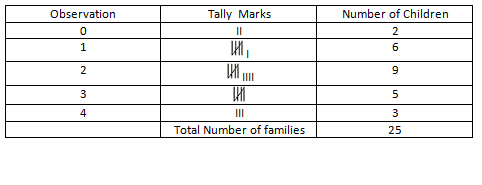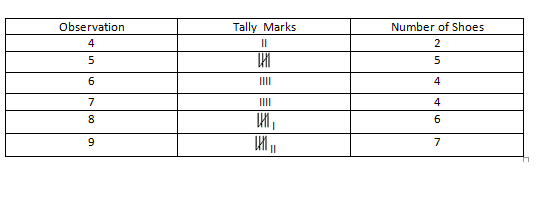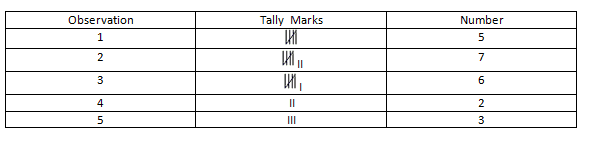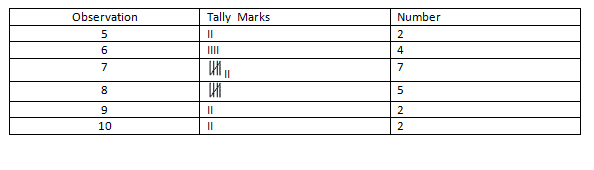RS Aggarwal Class 6 Solutions Chapter 22 - Data Handling

RS Aggarwal Class 6 Chapter 22 - Data Handling Solutions Free PDF

In this chapter, the students of class 6 will be taught about data. What is data and how to organize data, collect data and represent data on a bar graph or in a pictograph. Learn the concepts of data handling by solving the questions from RS Aggarwal for class 6. For solutions to the questions, students are advised to refer RS Aggarwal class 6 solutions chapter 22 data handling.

Exercise 22

Q1) Define the terms:

(i) Data (ii) Raw Data (iii) Array

(iv) Tabulation of data (v) Observations

(vi) Frequency of an observation (vii) Statistics

Ans. 1)

(i) Data: It refers to the information in the form of numerical figures.

The marks obtained by 5 students of a class in a unit test are 34, 45, 65, 67, 87.

We call it the data related to the marks obtained by 5 students of a class in a unit test.

(ii) Raw Data: Data obtained in the original form is called raw data.

(iii) Arrray: Arranging the numerical figures in an ascending or a descending order is called an array.

(iv) Tabulation of data: Arranging the data in a systematic form in the form of a table is called tabulation or presentation of the data.

(v) Observations: Each numerical figure in a data is called an observation

(vi) Frequency of an observation: The number of times a particular observation occurs is called its frequency.

(vii) Statistics: It is the science that deals with the collection, presentation, analysis and interpretation of numerical data.

Q2) The number of children in 25 families of a colony are given below:

2 , 0 , 2 , 4 , 2 , 1 , 3 , 3 , 1 , 0 , 2 , 3 , 4 , 3 , 1 , 1 , 1 , 2 , 2 , 3 , 2 , 4 , 1 , 2 , 2 .

Represent the above data in the form of a frequency distribution table.

Ans. 2)Q3) The sale of shoes of various sizes at a shop on particular day is given below:

6 , 9 , 8 , 5 , 5 , 4 , 9 , 8 , 5 , 6 , 9 , 9 , 7 , 8 , 9 , 7 , 6 , 9 , 8 , 6 , 7 , 5 , 8 , 9 , 4 , 5 , 8 , 7 .

Represent the above data in the form of a frequency distribution table.

Ans. 3)Q4) Construct a frequency table for the following:

3 , 2 , 5 , 4 , 1 , 3 , 2 , 2 , 5 , 3 , 1 , 2 , 1 , 1 , 2 , 2 , 3 , 4 , 5 , 3 , 1 , 2 , 3.

Ans. 4)Q5) Construct a frequency table for the following:

7 , 8 , 6 , 5 , 6 , 7 , 7 , 9 , 8 , 10 , 7 , 6 , 7 , 8 , 8 , 9 , 10 , 5 , 7 , 8 , 7 , 6.

Ans. 5)Q6) Fill in the blanks:

(i) Data means information in the form of _______ .

(ii) Data obtained in the _____ form is called raw data.

(iii) Arranging the numerical figures in an ascending or a descending order is called an ______ .

(iv) The number of times a particular observations occurs is called its ______ .

(v) Arranging the data in the form of a table is called ______ .

Ans. 6)

(i) numerical figures

(ii) original

(iii) array

(iv) frequency

(v) tabulation of data

Practise This Question

The materials which are attracted towards a magnet are called ______.